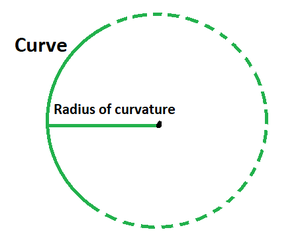Open in App
Not now

• Last Updated : 18 Apr, 2022

The curvature is that scalar value by which a curve deviates from being flat to a curve and from a curve back to a line. The reciprocal of the curvature is the radius of curvature and it is an imagined circle rather than a genuine form or figure. The radius of curvature is the radius of the approximate circle at a specific place. It is represented by the curvature vector length and denoted by the symbol R. It is defined as the distance between the vertex and the centre of curvature. As the curve advances, the radius changes.The radius of curvature of a curve is defined as the approximate radius of a circle at any given point or the vector length of a curvature. It exists for any curve with the equation y = f(x) with x as its parameter.

Radius of curvature, R =where,

dy/dx = first derivative of the function y = f(x),

d2y/dx2 = second derivative of the function y = f(x).

In case of polar coordinates r=r(θ), the radius of curvature is given bywhere,

K is the tangent vector function and curvature of the curve given by dT/ds,

r is the radius of curvature.

### Sample Problems

Problem 1. Find the radius of curvature for f(x) = 4x2 + 3x – 7 at x = 4.

Solution:

We have, y = 4x2 + 3x – 7 and x = 4.

dy/dx = 16x + 3

d2y/dx2 = 16

Using the radius of curvature formula, we get

R = (1 + (16x + 3)2)3/2/16

= (1 + 256x2 + 9 + 36x)3/2/16

= (256x2 + 36x + 10)3/2/16

Substitute the value x = 4.

R = (256 (4)2 + 36 (4) + 10)3/2/16

= (4096 + 144 + 10)3/2/16

= 27066/16

= 1691.625 units

Problem 2. Find the radius of curvature for f(x) = 3x2 + 3x – 2 at x = 1.

Solution:

We have, y = 3x2 + 3x – 2 and x = 1.

dy/dx = 6x + 3

d2y/dx2 = 6

Using the radius of curvature formula, we get

R = (1 + (6x + 3)2)3/2/6

= (1 + 36x2 + 9 + 36x)3/2/6

= (36x2 + 36x + 10)3/2/6

Substitute the value x = 1.

R = (36 (1)2 + 36 (1) + 10)3/2/6

= (36 + 36 + 10)3/2/6

= 742.54/6

= 123.75 units

Problem 3. Find the radius of curvature for f(x) = 3x3 – 2x + 7 at x = 2.

Solution:

We have, y = 3x3 – 2x – 2 and x = 2.

dy/dx = 9x2 – 2

d2y/dx2 = 18x

Using the radius of curvature formula, we get

R = (1 + (9x2 – 2)2)3/2/18x

= (1 + 81x4 + 4 – 36x2)3/2/18x

= (81x4 – 36x2 + 5)3/2/18x

Substitute the value x = 2.

R = (81 (2)4 – 36 (2) + 5)3/2/18 (2)

= (576 – 72 + 5)3/2/36

= 12305.26/36

= 512.71 units

Problem 4. Find the radius of curvature for f(x) = 5x3 – 3x2 + x at x = 1.

Solution:

We have, y = 5x3 – 3x2 + x and x = 1.

dy/dx = 15x2 – 6x + 1

d2y/dx2 = 30x – 6

Using the radius of curvature formula, we get

R = (1 + (15x2 – 6)2)3/2/(30x – 6)

= (1 + 225x4 + 36 – 180x2)3/2/(30x – 6)

= (225x4 – 180x2 + 37)3/2/(30x – 6)

Substitute the value x = 1.

R = (225(1)4 – 180(1)2 + 37)3/2/(30 (1) – 6)

= (225 – 180 + 37)3/2/24

= 742.54/24

= 30.93 units

Problem 5. Find the radius of curvature for the curve f(x) = x2.

Solution:

We have the curve, y = x2

dy/dx = 2x

d2y/dx2 = 2

Using the radius of curvature formula, we get

R = (1 + (2x)2)3/2/2

= (1 + 4x2)3/2/2

= (1 + 4y)3/2/2

Problem 6. Find the radius of curvature for the curve f(x) = sin x.

Solution:

We have the curve, y = sin x.

dy/dx = cos x

d2y/dx2 = – sin x

Using the radius of curvature formula, we get

R = – (1 + (cos x)2)3/2/sin x

= (1 + cos2 x)3/2/sin x

= (1 + cos2 x)3/2/y

Problem 7. Find the radius of curvature for the curve f(x) = ex.

Solution:

We have the curve, y = ex.

dy/dx = ex

d2y/dx2 = ex

Using the radius of curvature formula, we get

R = – (1 + (ex)2)3/2/ex

= (1 + e2x)3/2/ex

= (1 +y2)3/2/y

My Personal Notes arrow_drop_up
Related Articles